## 48) Subtraction

Ten Plus Bonds: Tic-Tac-Toe

### Mathematics

Develop fluency recalling two-part, ten plus bonds of 20 to find the missing part in subtraction equations.

### Language

• subtract
• equals
• part-part-whole

### Core Activity

There are two activity boards

• Section One: the student knows (from what they spun) the single-digit part. For example, 3. They have to look for the ten plus part that builds 20, which is 17. Section Two is the reverse.
• Section Two: the student knows (from what they spun) the ten plus part. For example, 17. They have to look for the part that builds 20, which is 3.

For example, Section One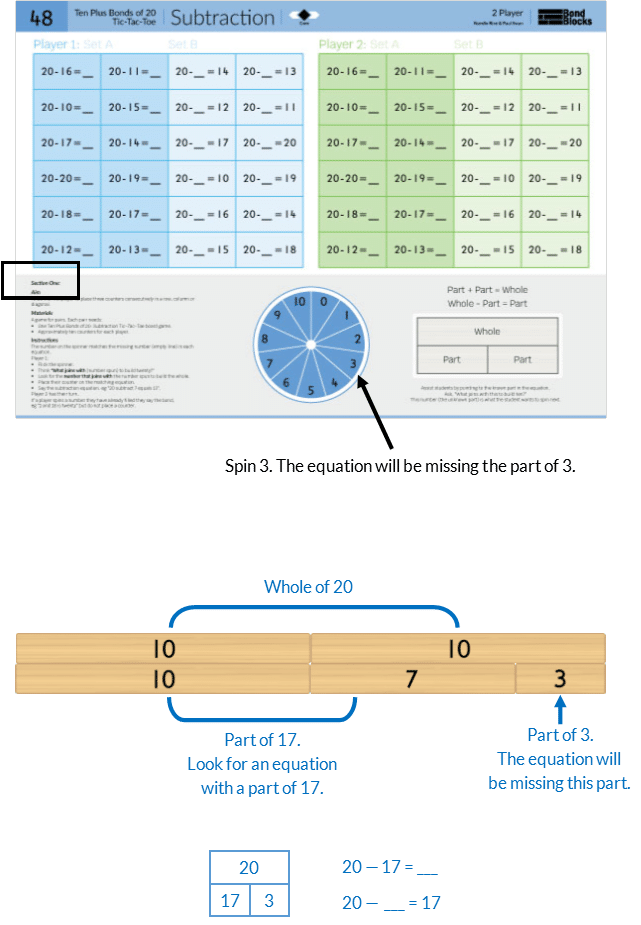For example, Section Two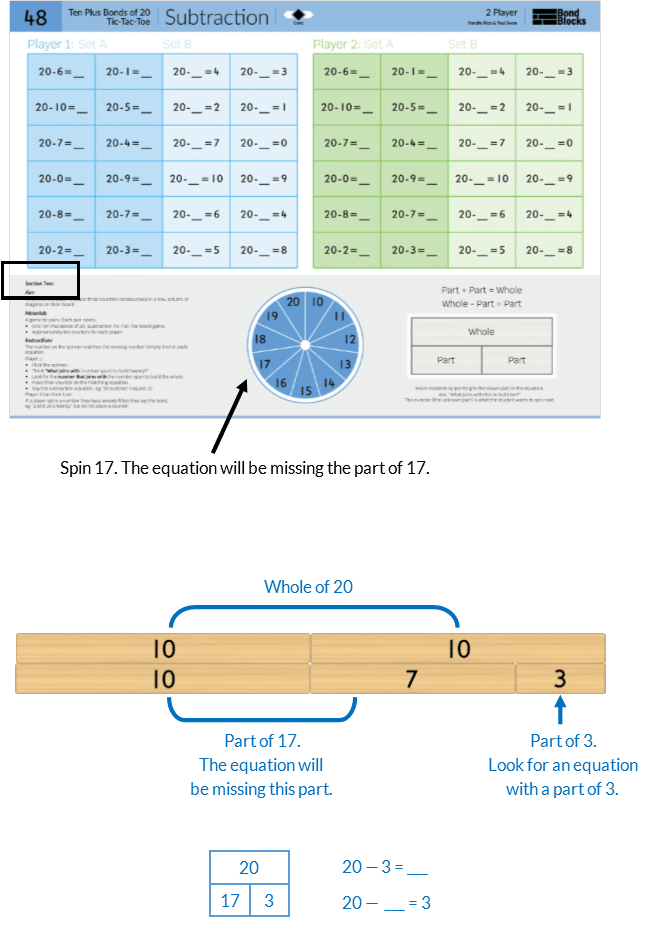## Differentiation

### A little easier

##### Set A then Set B

The equations on each activity board have been colour coded into two sets.

•  Set A: the missing part in the the equation is in the typical answer position. For example, 20 – 16 = ?
• Set B: the missing part in the equation is in the atypical position position. For example, 20 – ? = 16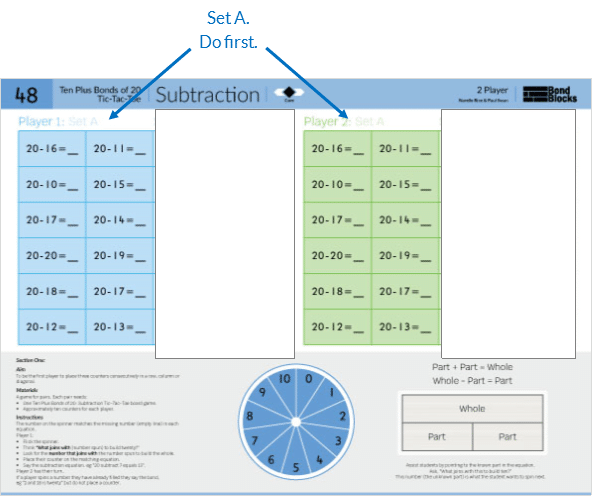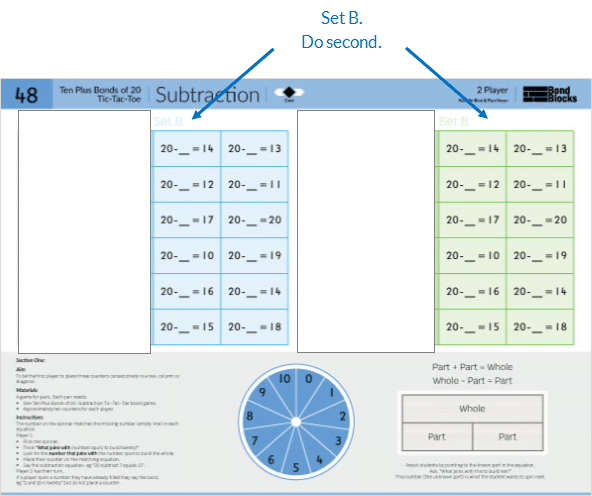• Play with only Set A. Cover Set B. The player who places the most number of counters in 3 minutes wins.
• Once students have developed fluency with Set A move on to Set B. Play with only Set B. Cover Set A.
• When students are fluent with Set B play with both sets at the same time.

Repeat this for the activity board in Section Two.

##### Use blocks

Students use Bond Blocks to support calculation.

• First, represent the whole of 20.
• Next, represent the part spun.
• Then, represent the remaining part that joins with the part spun.
• Look for the number made by the remaining part on the game board.
• Place their counter on the matching equation.
• Say the subtraction equation.

Blocks are placed using a part-part-whole representation. Organise the ten plus part on the left and the remain part on the right. This supports the representation used in the previous activity and also makes clear the related bond of 10.

For example, in Section One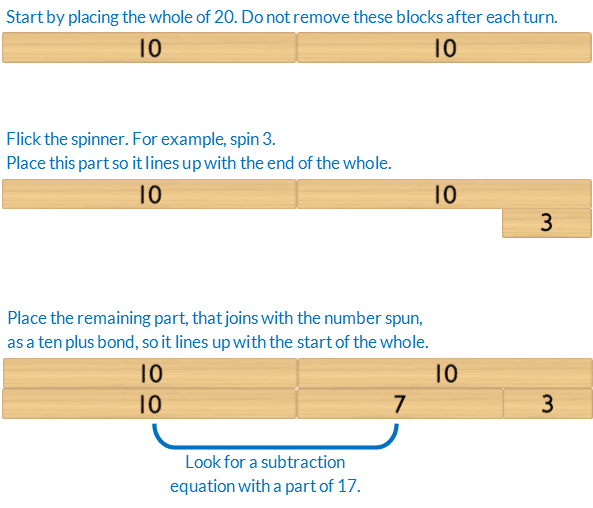For example, in Section Two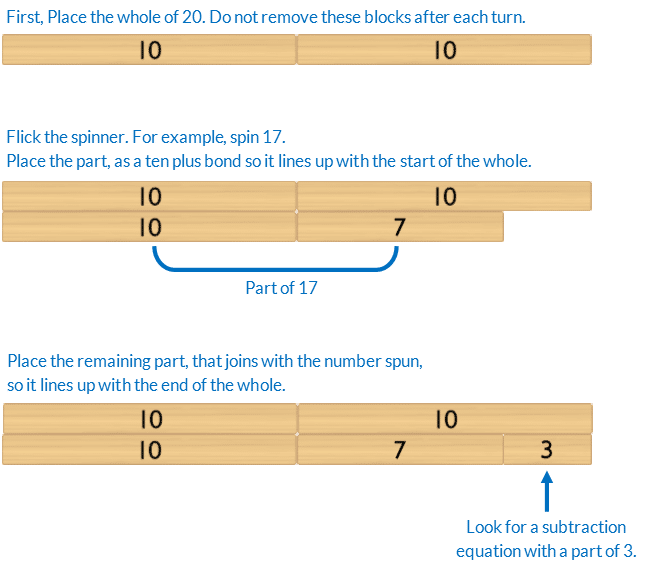As students become fluent using the blocks to represent the subtraction they will want to leave the part of 10 under the whole after each turn. This increases the efficiency of using the blocks. Encourage this.

• Draw attention to the bond of 10 the student is focusing on. Ten plus bonds of 20 are just extended bonds of 10. It is inefficient to think of them as separate bonds. Encourage verbalisation of mathematical relationships and reasoning.
• The aim is to remove the need for blocks as students progress towards the abstract use of numbers.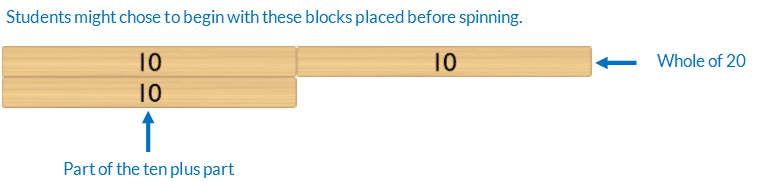### A little harder

##### Set A and Set B

Start with the activity board in Section One. Play with both Set A and Set B at the same time.

Repeat for the activity board in Section Two.

### Progression

In the next activity students build on subtraction equations with a missing part to find missing parts and wholes for addition and subtraction equations for ten plus bonds of 20. Go to

##### Activity 49

Ten Plus Bonds: Missing Number Equations, Tic-Tac-Toe